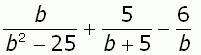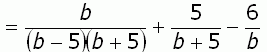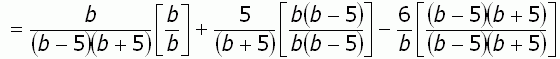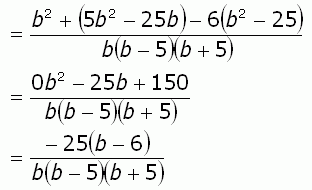SEARCH HOMEMath Central Quandaries & QueriesQuestion from Jessica, a student: I can't figure out this problem. This is a Algebra problem.  first it says to  Perform the indicated operation and simplify. b/ b2-25 + 5/b+5 -6/b  Need to find the LCD b2 is a b to the 2nd power and the answer looks like this -25(b-6)/b(b+5)(b-5)Hi Jessica. I've re-written the starting point:Can you see that b2 - 25 is a difference of squares? That means the first term is a perfect square, the second term is a perfect square and there is a minus between them. Whenever you see this, you know it factors into the sum of the roots times the difference of the roots:Now that all the denominators are broken into their factors, you can readily find a common denominator by multiplying (b-5)(b+5)(b). So the first term needs a b in the denominator, so you multiply it by b/b, the second term needs two factors: b and b-5, so multiply by both, top and bottom, and so on.Finally, multiply it out and simplify:I am showing the same answer you were given. Can you find the error you made?

Hope this helps,
Stephen La Rocque.Math Central is supported by the University of Regina and The Pacific Institute for the Mathematical Sciences.